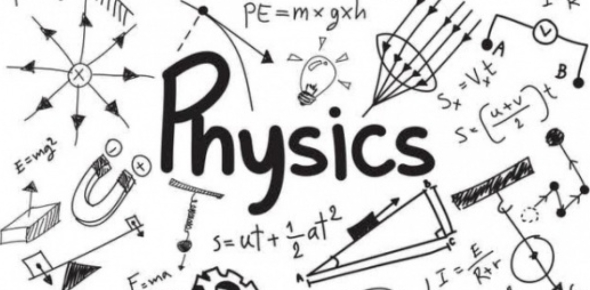# Quite Tricky And Difficult Physics Trivia Questions

15 Questions | Attempts: 2004SettingsCan you give the correct answers about each of these physics questions

• 1.
Which scientific term is best defined as an electrically charged atom?
• A.

Waterspout

• B.

Ion

• C.

Beaver Dam

• D.

Barrel

• 2.
What is a space which is entirely devoid of matter called in science terms?
• A.

Lumen

• B.

Blank World

• C.

Transparent

• D.

Vacuum

• 3.
What is the distance between two peaks of a wave called?
• A.

Atomic Weight

• B.

Wavelength

• C.

Wave Dip

• D.

Aurora

• 4.
What is the rate at which something moves called?
• A.

Orbit

• B.

Momentum

• C.

Speed

• D.

Mileage

• 5.
A unit of electromotive force is called what?
• A.

Chain

• B.

Eclipse

• C.

• D.

Volt

• 6.
What unit of measurement is one-thousandth of a gram?
• A.

Aqueduct

• B.

Methane

• C.

Milligram

• D.

Kilometer

• 7.
What is water in its solid form called?
• A.

Photon

• B.

Ice

• C.

Vapor

• D.

Viscosity

• 8.
A unit for measuring pressure is also known as what?
• A.

Thermometer

• B.

Mineral

• C.

Pounds Per Square Inch (Psi)

• D.

Analgesic

• 9.
What is the irregular and instantaneous motion of air called?
• A.

Sun Storm

• B.

Turbulence

• C.

Air Pocket

• D.

Fauna

• 10.
What does splitting a laser light into two beams through a silver mirror make?
• A.

Digital Images

• B.

Static Electricity

• C.

Nuclear Power

• D.

Hologram

• 11.
Which term describes flying at six times the speed of sound?
• A.

Muck 6

• B.

Mach 1

• C.

Mach 6

• D.

Ascap 2

• 12.
What is another name for a volt-ohm meter?
• A.

Laser Counter

• B.

Scope

• C.

Wire Tracer

• D.

VOM

• 13.
Which abbreviation refers to a term that literally means "below red"?
• A.

IR

• B.

ROTC

• C.

CD

• D.

ROM

• 14.
The four engines of a Boeing 747 jet produce 188,000 pounds (85,275 kilograms) of what?
• A.

Emissions

• B.

Thrust

• C.

Human Waste

• D.

Rock

• 15.
Which of these is a property of a marble?
• A.

It Will Sink In A Glass Of Water

• B.

It Is Fuzzy

• C.

It Will Float In A Glass Of Water

• D.

It Is Soft

## Related TopicsBack to top
×

Wait!
Here's an interesting quiz for you.